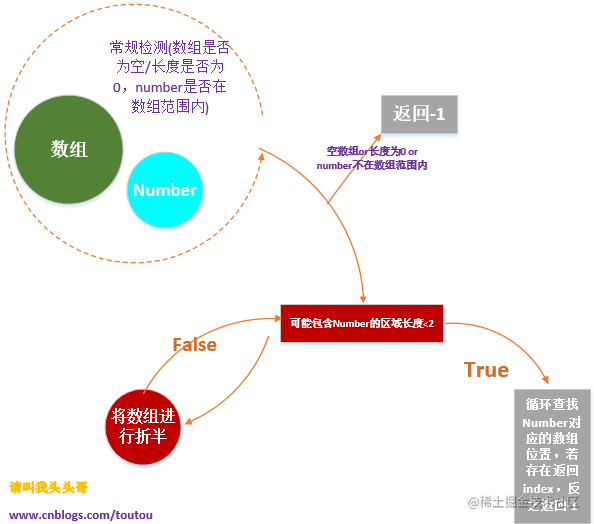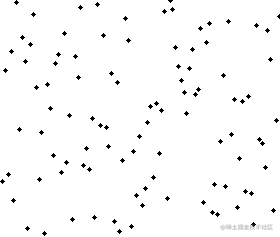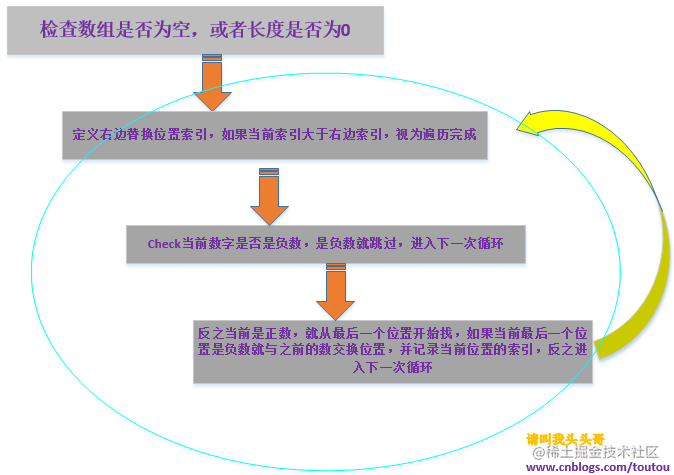# 算法面试题## v算法百科

----算法百科摘自百度百科(ps:不摘点介绍性的文字在这，直接开门见山的来题目。感觉有点duangduang的。性急的可以直接跳过此处！)

## v算法题目

• 第一题：在一个已经排序的Int数组中，查找某个number，如果存在这个number，返回在数组的位置，反之返回-1 查看博主面试答案
• 第二题：{"12,bob","3,sky","6,cool","1,good","22,go"}，按元素第一列排序 查看博主面试答案
• 第三题：字符串数组去除重复项 查看博主面试答案
• 第四题：将一个未排序的整形数组进行归置，数组中的负数移动到数组的左边，正数移动到数组的右边。0不动(ps:我一开做这题就打算用无脑排序搞定，结果我才开始写，面试官就说了"这里需要提示一下，移动后的数组只是负数在左，正数在右。不一定非要用传统的排序，如果不是传统的排序可以加分。") 查看博主面试答案

## v参考答案

### 第一题

``````//------------------------------------------------------------------------------
// <copyright file="Runner.cs" company="CNBlogs Corporation" owner="请叫我头头哥">
//     原博文地址： http://www.cnblogs.com/toutou/
//------------------------------------------------------------------------------
namespace TestApp
{
using System;
using System.Collections.Generic;
using System.Text.RegularExpressions;
class Runner
{
static void Main(string[] args)
{
int[] arr = { 3, 5, 6, 7, 8, 9, 14, 23, 45, 56, 63, 72, 87, 91, 92, 93, 95, 97, 98, 534, 555, 676, 878, 988, 1365 };
int number = 555;
int result = Search(arr, number);
Console.WriteLine(result);
}
public static int Search(int[] arr, int number)
{
int result = 0;
if (arr == null || arr.Length == 0 || number > arr[arr.Length - 1] || number < arr)
{
result = -1;
}
else
{
result = Bisearch(arr.Length - 1, arr, number);
}
return result;
}
public static int Bisearch(int endIndex, int[] arr, int number, int startIndex = 0)
{
int result = 0;
if ((endIndex - startIndex) < 2)
{
for (int i = startIndex; i <= endIndex; i++)
{
if (arr[i] == number)
{
result = i;
break;
}
else
{
result = -1;
}
}
}
else
{
if (arr[startIndex] <= number && number <= arr[(endIndex + startIndex) / 2])
{
Bisearch((endIndex + startIndex) / 2, arr, number, startIndex);
}
else
{
Bisearch(endIndex, arr, number, (endIndex + startIndex) / 2 + 1);
}
}
return result;
}
}
}### 第二题

``````namespace TestApp
{
using System;
using System.Text.RegularExpressions;
class Program
{
static void Main(string[] args)
{
string[] source = new string[] { "12,bob", "3,sky", "6,cool", "1,good", "22,go" };
for (int i = 0; i < source.Length; i++)
{
for (int j = i; j < source.Length; j++)
{
if (Convert.ToInt32(Regex.Match(source[i], @"\d+").Value) > Convert.ToInt32(Regex.Match(source[j], @"\d+").Value))
{
string temp = source[i];
source[i] = source[j];
source[j] = temp;
}
}
}
for (int i = 0; i < source.Length; i++)
{
Console.WriteLine(source[i]);
}
}
}
}### 第三题

``````namespace TestApp
{
using System;
class Program
{
static void Main(string[] args)
{
string[] source = { "aaa", "bbb", "aaa", "ccc", "bbb", "ddadd", "ccc", "aaa", "bbb", "ddd" };
foreach (var item in ArrDistinct(source))
{
Console.WriteLine(item);
}
}
public static String[] ArrDistinct(string[] source)
{
if (source != null && source.Length > 0)
{
Array.Sort(source);
int size = 1;
for (int i = 1; i < source.Length; i++)
if (source[i] != source[i - 1])
size++;
string[] tempArr = new string[size];
int j = 0;
tempArr[j++] = source;
for (int i = 1; i < source.Length; i++)
if (source[i] != source[i - 1])
tempArr[j++] = source[i];
return tempArr;
}
return source;
}
}
}

``````//因为这题的主要考点在字符串数组去重，所以排序我就没太在意，直接用的系统排序。当然，这样是很不专业的。不过如果有必要的话，这里也可以先用算法排序字符串，然后再用我这个办法。 至于算法排序字符串，我能想到的比较"卑鄙"的办法就只有先将字符串数组转成char再转成int数组，
Array.ConvertAll<string, int>(strArray, s => int.Parse(s));
//再排序，大神你有更好的办法？有就不要藏着掖着了，来吧！

### 第四题

``````public int[] IntArrSort(int[] source)
{
if (source == null || source.Length == 0)
return source;
int rightIndex = source.Length - 1, tempNumber = 0;
for (int i = 0; i < source.Length; i++)
{
if (i > rightIndex)
break;
if (source[i] <= 0)
{
continue;
}
else if (source[i] > 0)
{
for (int j = rightIndex; j >= 0; j--)
{
if (source[j] < 0)
{
tempNumber = source[j];
source[j] = source[i];
source[i] = tempNumber;
rightIndex = j;
break;
}
}
}
}
return source;
}## v博客总结

各位道友，以上所有的算法面试题都是我平常在面试中积累下来的，出场率比较高的我都列出来了(出场率只是相对我面试的经历而言)。